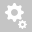##Recently viewed tickets# Composite section analysis

Question:

Hi

I have created a grillage model for a single span bridge using precast concrete composite girder (Super T girders), taking into account of the construction sequence where the deck pour is done after the precast girders are installed at the support.
The model file is attached.
I have two questions related to the results and I hope you can help:

1.  How can I get the element properties of the composite member, in particular the Elasticity of superstructure's at each construction stage? When I use the results tables, it gave me "-" (see Capture 1).

2.  From the analysis, the deflection of the precast Super T girder after deck is pour (Dead load only) as stage 3 construction (Cast Main Deck Stage 1) is about 0.015m (see Capture 2).
However, a simple calulation based on deflection of a simply supported beam, the expected deflection is about 0.029m, with on the following parameters.
L = 39.65m,  I = 0.266m4, E = 3.48E7 kN/m2 and Area of deck = 0.339m2 (w = 8.48 kN/m)

I wonder what have I done wrong?
Looking forward to hear from you soon.

Appreciate for the quick respond, thanks!

Question: How can I get the element properties of the composite member, in particular the Elasticity of superstructure's at each construction stage? When I use the results tables, it gave me "-" (see Capture 1).
Answer: Composite section property are not visible in the results table of "Element property at each stage". Its property is only visible in .OUT file formed after analysis.

Question2: rom the analysis, the deflection of the precast Super T girder after deck is pour (Dead load only) as stage 3 construction (Cast Main Deck Stage 1) is about 0.015m (see Capture 2).
However, a simple calulation based on deflection of a simply supported beam, the expected deflection is about 0.029m, with on the following parameters.
L = 39.65m,  I = 0.266m4, E = 3.48E7 kN/m2 and Area of deck = 0.339m2 (w = 8.48 kN/m)
Answer: This is because the deck is activated in stage 3 and section has become composite at the same stage when deck is casted as shown below.Therefore, the composite Iyy = 0.5422, Area of deck: 1.8*0.2= 0.36 m2 and density of C40 is 23.54, (w= 8.474 kN/m) as shown below.Therefore, the deflection calculated from above data is 0.0144 m which is approximately equal to midas.

To avoid this apply the wet concrete uniform load before activating the deck part. And deactivate the wet concrete load in next stage and activate the deck part in that construction stage.

1) So, first make wet concrete load case and load group. Apply uniform load of -8.474 kN/m to girder1 group.2) Activate wet concrete load group in CS3 "casting deck 1" and remove the dummy transverse structural group elements named "Deck main Slab-1."3) Then create a new construction stage 4 (Activate deck 1) and activate deck main slab-1 structure group. Deactivate the wet concrete load group in CS4 and activate the composite section in construction stage in CS4 as shown below.This is correct method to activate the different parts of composite section and application of their wet concrete load.
Also adjust the age and stage duration of each stage according to project requirement.

After improving this, deflection for mid girder at CS3 "Casting Deck slab 1" due to wet concrete is 0.029m which is approximately equal to hand calculated value.Similarly, do this for other deck slab activation.
The model is attached for further review.
Creation date: 6/8/2021 1:52 PM (jatin2010)      Updated: 6/8/2021 1:52 PM ()